# 一、学习总结

## 1、树结构思维导图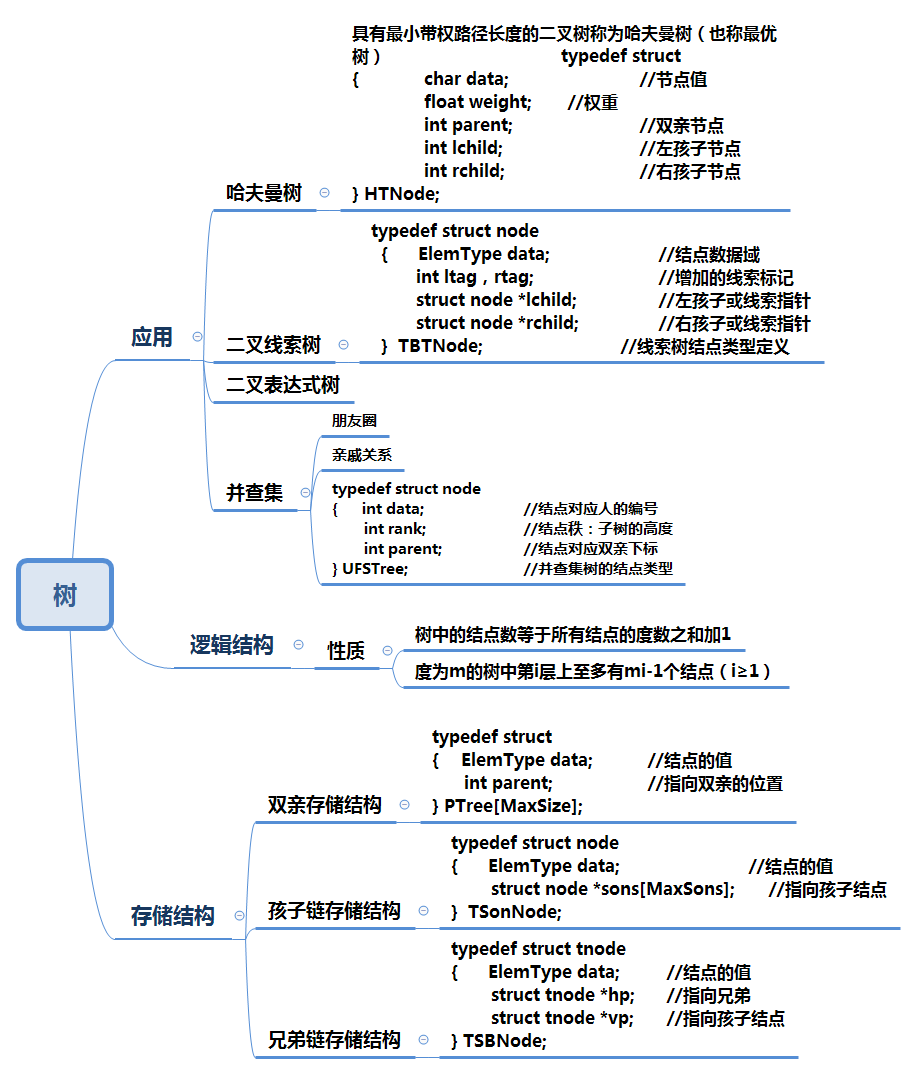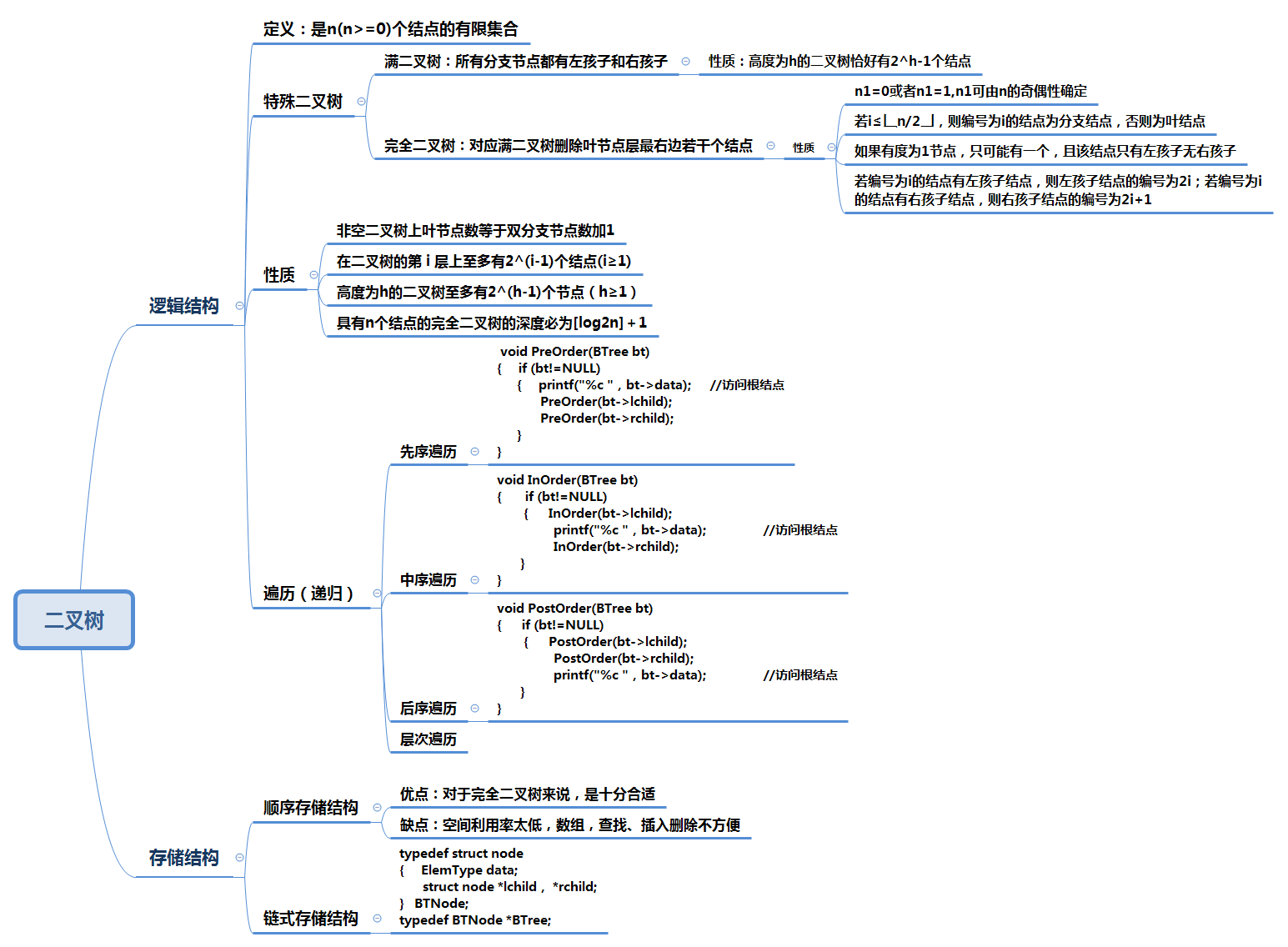## 2、树结构学习体会

• 树这一节遇到最大的困难就是递归不能灵活的运用，总是想用链表那里的知识解决，做了一大堆，程序崩溃也找不到问题出在哪里。

# 二、PTA实验作业

## 题目1:表达式树

### 1、设计思路

void InitExpTree(BTree &T,string str)
{
定义i做下标
定义栈s用于存放树的节点数据
定义字符栈p用来存放符号
入栈#用作空栈标识

while (str[i])
{
判断str[i]不是字符
{
创建树T并申请空间
将str中的数据赋给树T
左右子树初始化
T中数据入栈s
}
否则
{
switch判断字符
{
"<"时
数据入栈p
i++;
break;
"="时
p栈顶元素出栈;
i++;
break;
">"时
创建新节点T
将p栈顶元素赋给T
p栈顶元素出栈
将s的栈顶元素赋给T的右孩子
s栈顶元素出栈
if(s不为空)
{
将s栈顶元素赋给左孩子
s栈顶元素出栈
}
T元素入栈s
break;
}
}
}
while p栈顶元素不为'#'
{
创建新节点T
将p栈顶元素赋给T
p栈顶元素出栈
将s的栈顶元素赋给T的右孩子
s栈顶元素出栈
if(s不为空)
{
将s栈顶元素赋给左孩子
s栈顶元素出栈
}
T元素入栈s
}
double EvaluateExTree(BTree T)
{
定义a b两个运算变量
判断T的左右孩子都不存在
返回 T->data-'0';
a=EvaluateExTree(T->lchild);
b=EvaluateExTree(T->rchild);
switch T此时的元素
{
'+'时
返回a+b;
break;
'-'时
返回a-b;
break;
'*'时
返回a*b;
break;
'/'时
if(b==0)
打印"divide 0 error!"
否则返回a/b;
break;
}
}



### 2、代码截图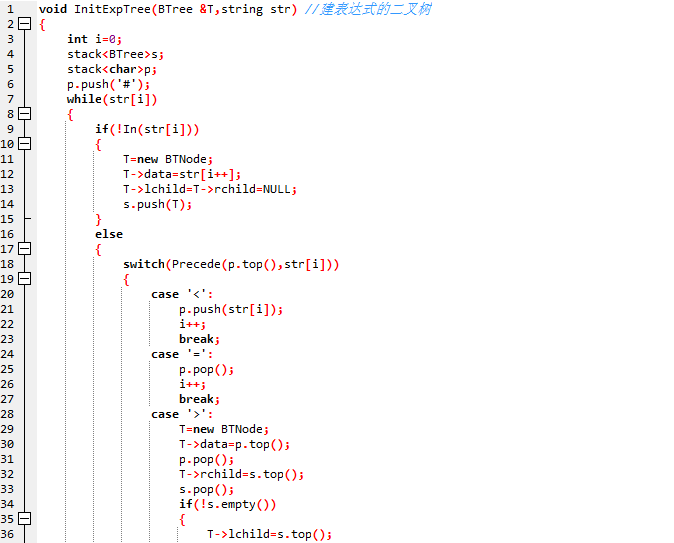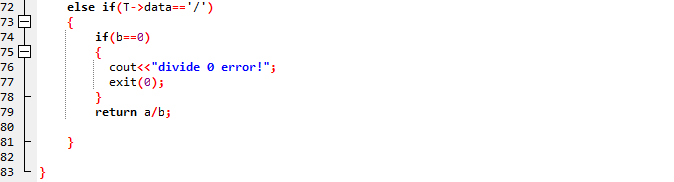### 3、PTA提交列表说明## 题目2:修理牧场

### 1、代码截图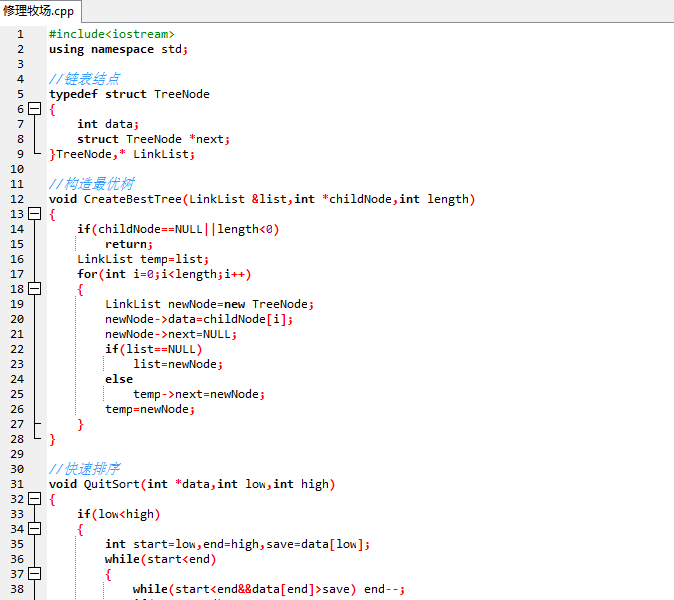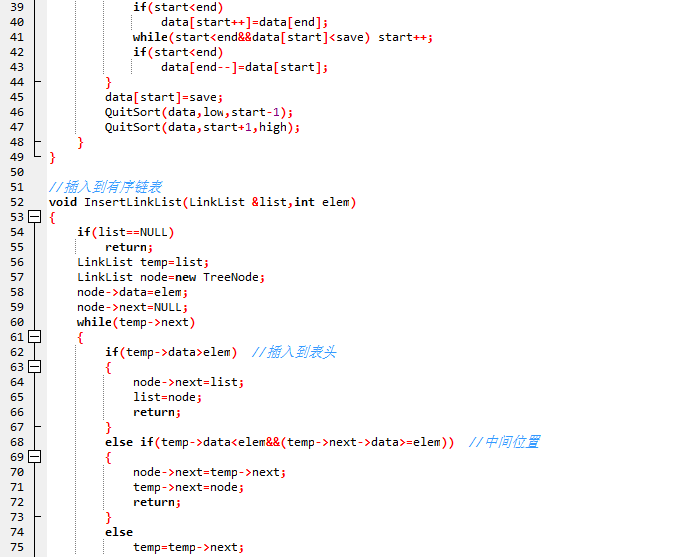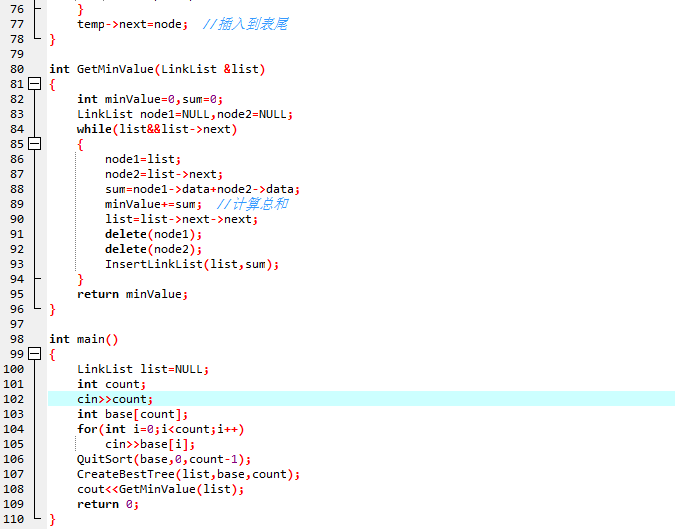### 2、PTA提交列表说明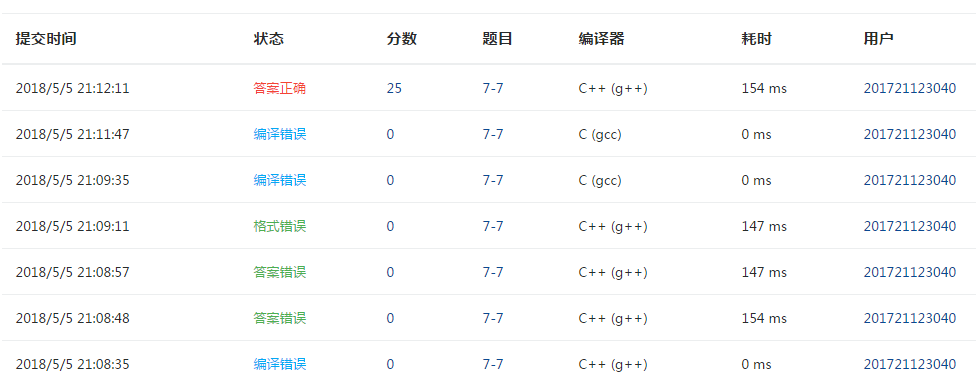## 题目3:求二叉树高度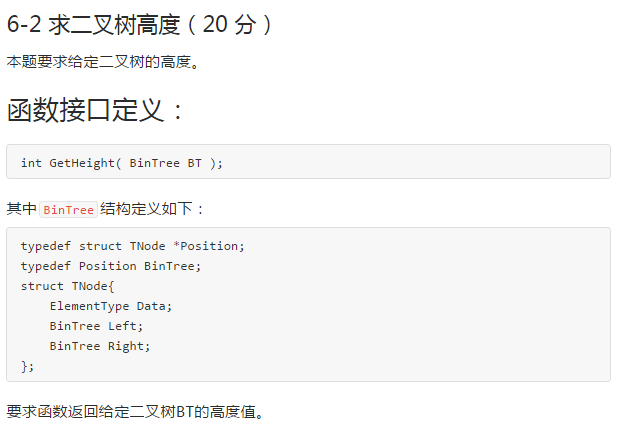### 1、设计思路

定义左子树高度、右子树高度变量



### 2、代码截图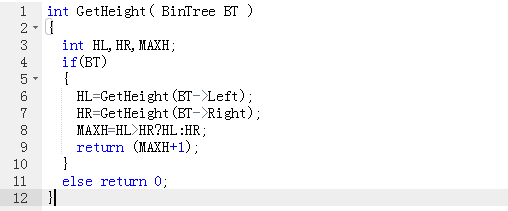### 3、PTA提交列表说明# 三、截图本周题目集的PTA最后排名

## 1、PTA排名# 四、 阅读代码

## 修理牧场优秀代码

### 1、代码

---------------------------------优先队列做法-------------------------------
#include <cstdio>
#include <queue>

using namespace std;

priority_queue<int, vector<int>, greater<int> > q;

int main() {
int n, m;

scanf( "%d", &n );

for( int i = 0; i < n; i++ ) {
scanf( "%d", &m );
q.push( m );
}

int sum = 0;

while( q.size() > 1 ) {
int first = q.top();
q.pop();

int second = q.top();
q.pop();

sum += first + second;
q.push( first + second );
}

printf( "%d\n", sum );

return 0;
}

--------------------------数组做法---------------------------
# include <stdio.h>
# include <malloc.h>

int main ()
{
int i, m, num, t;
int val;
int cost=0;

scanf ("%d", &num);
int *a=(int*)malloc(sizeof(int)*num);

for (i=0;i<num;i++)
scanf ("%d", &a[i]);
while (i!=1)
{
m=i-1;
for (int j=0;j<i;j++)
for (int k=0;k<i; k++)
if (a[j]>a[k])
{
t=a[j];
a[j]=a[k];
a[k]=t;
}
//for (int z=0;z<i;z++)
//  printf("%d ",a[z]);
//printf ("\n");
a[m-1]=a[m-1]+a[m];
cost=cost+a[m-1];
//printf("%d\n",a[m]);
i--;
}
printf ("%d\n", cost);
return 0;
}


## 学习内容：

### 优先队列：它的“优先”意指取队首元素时，有一定的选择性，即根据元素的属性选择某一项值最优的出队~

优先级队列 是不同于先进先出队列的另一种队列。每次从队列中取出的是具有最高优先权的元素
优先队列的类定义
优先队列是0个或多个元素的集合,每个元素都有一个优先权或值,对优先队列执行的操作有： 查找; 插入一个新元素; 删除.

### 给出一行字符串，求出其原编码需要的编码长度和哈夫曼编码所需的长度，并求其比值

#include<stdio.h>
#include<string.h>
#include<ctype.h>
#include<functional>
#include<queue>
using namespace std;
#define M 1000050
char str[M];
int list;
priority_queue< int,vector<int>,greater<int> >que;
int main()
{
int ans,sum;
int i,a,b,c;
while(scanf("%s",str),strcmp(str,"END")){
memset(list,0,sizeof(list));
for(i=0;str[i];i++){
if(isalpha(str[i]))
list[str[i]-'A']++;
else
list++;
}
sum=i*8;ans=i;c=0;
for(i=0;i<27;i++){
if(list[i]){
que.push(list[i]);
c++;
}
}
if(c>1){ans=0;//注意只有一种字符的情况
while(que.size()!=1){
a=que.top();
que.pop();
b=que.top();
que.pop();
ans+=a+b;
que.push(a+b);
}
while(!que.empty())//使用后清空队列
que.pop();
}
printf("%d %d %.1f\n",sum,ans,1.0*sum/ans);
}
return 0;
}


# 五、代码Git提交记录截图posted @ 2018-05-05 19:57  limb171004  阅读(279)  评论(2编辑  收藏  举报